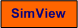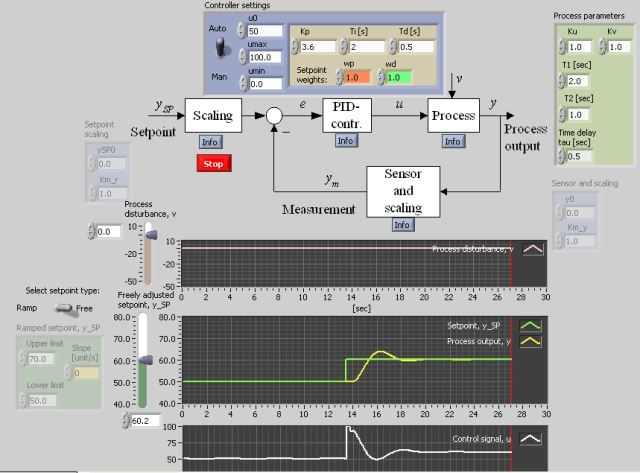# Reducing Control Kicks in PID Controller

Snapshot of the front panel of the simulator:• What is needed to run the the simulator? Read to get most recent information!
• Tips for using the simulator.
• The simulator: . The simulator runs immediately after the download by clicking Open in the download window. Alternatively, you can first save a copy of the exe-file on any directory (folder) on your PC and then run the exe-file, which starts the simulator.

## Description of the system to be simulated

In this simulator a control system for a process represented by a transfer function model is simulated. The process model is two time constant terms and a time delay in series. The controller is a PID controller with the possibility for the user to select reduced setpoint weight in the proportional and in the serivative terms.

The derivative term is given byer

ud = KpTd d(wdySP-y)/dt

where wd is the weight of value either 1 or 0 (values between 1 ans 0 are not used in practice). Normally wd is 1. With wd equal to 0 the setpoint is effectively removed from the derivative term.

In some PID controllers the user can select a reduced setpoint weight also in the proportional term:

up = Kp(wpySP-y)

where the weight wp can be e.g. 0.3 (normally 1).

Reduced setpoint weight implies that abrupt setpoint changes (as step changes) are not propagated to the control signal. This results in a smoother control signal, but it also causes a somewhat more sluggish control system as far as setpoint tracking is concerned, which may be unfortunate when the controller is a secondary controller in a cascade control system.

An alternative way to achieve a smoother control signal is to simply use a smoother setpoint signal, e.g. changing the setpoint between two values as a ramp in stead of as a step.

## Aims

The aims of this simulator is to observe the benefits and drawbacks of using reduced setpoint weights in the proportional term and the derivative term of a PID controller, and to see that ramping of the setpoint can be an alternative to reduced setpoint weighting.

## Motivation

In many commercial controllers the user can select reduced setpoint weight in the proportional and in the derivative term. It is important to be aware the benefits and drawbacks of such reduced weighting, and to know about the alternative solution of choosing smooth setpoint changes, a ramped change between two setpoint values.

If the controlled process has default parameter values (i.e. Ku=1, Kv=1, T1=2, T2=1, tau=0.5) proper PID settings are

Kp=3.6; Ti=2.0; Td=0.5

(as found using the Ziegler-Nichols' closed loop method). These settings may be used in the tasks below.

Start the simulator.

1. Reduced setpoint weight in the D term:
1. Change the setpoint as a step (e.g. from 50% to 60%). What is the consequence for the response in the control variable of using reduced setpoint weight wd = 0 in stead of the normal value of 1?
2. Change the setpoint as a step (e.g. from 50% to 60%). Does a reduced weight wd have any impact on the setpoint tracking? Why/Why not?
3. Change the process disturbance as a step Endre forstyrrelsen som et sprang (e.g. from 0 to -40). Does the reduced weight wd have any impact on the disturbance tracking of the control system. Why/Why not?

2. Reduced setpoint weight in the P term:
1. Change the setpoint as a step (e.g. from 50% to 60%). What is the consequence for the response in the control variable of using setpoint the reduced weight wp = 0,3 in stead of the normal value of 1?
2. Change the setpoint as a step (e.g. from 50% to 60%). Does a reduced weight wp have any impact on the setpoint tracking? Why/Why not?
3. Change the process disturbance as a step Endre forstyrrelsen som et sprang (e.g. from 0 to -40). Does the reduced weight wd have any impact on the disturbance tracking of the control system. Why/Why not?

3. Using ramped setpoint changes: Set the setpoint weights wp and wd to 1. Is the control signal smoother at a ramped setpoint change than at a stepwise change?

[SimView] [TechTeach]

Updated 17. January 2008. Developed by Finn Haugen. E-mail: finn@techteach.no.